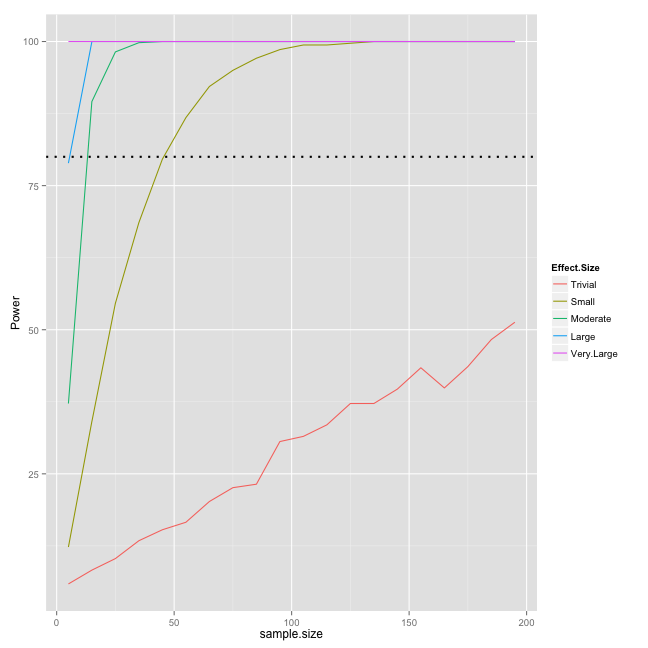## Wednesday, February 12, 2014

### “Power” to detect statistically significant effects based on sample size and magnitudes of effects

“Power” to detect <strong><em>statistically significant</em></strong> effects based on sample size and magnitudes of effects

# “Power” to detect statistically significant effects based on sample size and magnitudes of effects

I was going through magnitude-based inferences materials by Will Hopkins and I am playing with R simulations. I wanted to see how many times I am able to detect statisticaly significant effects (p<0.05) depending on magnitude of effects (expressed as Cohen's D, and using Will Hopkins levels) and sample sizes.

What I did is created a baseline group (mean = 100, SD = 10), and 5 more groups based on magnitude of difference (Trivial, Small, Medium, Large, Very Large) and repeated this for different number of subjects. Then I calculated p values using t test between baseline group and 5 other groups for each number of subjects. Then I repeated this process 1000 times and counder significant effects (p<0.05).

The result is the table showing how many times (percentage) in those 1000 resampling I was able to detect statisticly significant effect depending on the number of subjest of magnitude of change (from baseline group).

Here is the code and the resulting table:

``````effect.magnitudes <- c(0, 0.2, 0.6, 1.2, 2, 4)
effect.names <- c("Baseline", "Trivial", "Small", "Moderate", "Large", "Very.Large")
subjects.list <- seq(from = 5, to = 200, by = 10)

p.value <- matrix(0, nrow = length(subjects.list), ncol = length(effect.names) -
1)
colnames(p.value) <- effect.names[-1]
rownames(p.value) <- subjects.list
alpha <- 0.05

re.sampling <- 1000
significant.effects <- matrix(0, nrow = length(subjects.list), ncol = length(effect.names) -
1)
colnames(significant.effects) <- effect.names[-1]
rownames(significant.effects) <- subjects.list

for (k in 1:re.sampling) {
for (j in seq_along(subjects.list)) {
subjects <- subjects.list[j]
standard.deviation <- 30
sample.mean <- 100
dataSamples <- matrix(0, nrow = subjects, ncol = length(effect.magnitudes))

for (i in seq_along(effect.magnitudes)) dataSamples[, i] <- rnorm(n = subjects,
mean = sample.mean + standard.deviation * effect.magnitudes[i],
sd = standard.deviation)

colnames(dataSamples) <- effect.names
dataSamples <- as.data.frame(dataSamples)

p.value[j, 1] <- t.test(dataSamples\$Baseline, dataSamples\$Trivial)\$p.value
p.value[j, 2] <- t.test(dataSamples\$Baseline, dataSamples\$Small)\$p.value
p.value[j, 3] <- t.test(dataSamples\$Baseline, dataSamples\$Moderate)\$p.value
p.value[j, 4] <- t.test(dataSamples\$Baseline, dataSamples\$Large)\$p.value
p.value[j, 5] <- t.test(dataSamples\$Baseline, dataSamples\$Very.Large)\$p.value
}

significant.effects <- significant.effects + (p.value < alpha)
}

significant.effects <- significant.effects/re.sampling * 100
``````
Trivial Small Moderate Large Very.Large
5 6 12 37 79 100
15 8 34 90 100 100
25 10 55 98 100 100
35 13 69 100 100 100
45 15 80 100 100 100
55 17 87 100 100 100
65 20 92 100 100 100
75 23 95 100 100 100
85 23 97 100 100 100
95 31 99 100 100 100
105 32 99 100 100 100
115 34 99 100 100 100
125 37 100 100 100 100
135 37 100 100 100 100
145 40 100 100 100 100
155 43 100 100 100 100
165 40 100 100 100 100
175 44 100 100 100 100
185 48 100 100 100 100
195 51 100 100 100 100

As can be seen from the table, the number of subjects needed to get over 80% statistical power (chances to detect real effect) for Trivial is well above 300 (I did regression to find it out), for Small around 50, for Moderate probably around 10, for Large over 5 and Very Large effects are always detected (minimum 5 subjects). Someone please correct me if I am wrong here

Here is the graph of the above table, but to graph it in ggplot we need to reshape it

``````library(reshape2)
library(ggplot2)

significant.effects <- as.data.frame(significant.effects)
significant.effects <- data.frame(sample.size = as.numeric(rownames(significant.effects)),
significant.effects)
rownames(significant.effects) <- NULL

significant.effects.long <- melt(significant.effects, id.var = "sample.size",
value.name = "Power", variable.name = "Effect.Size")

gg <- ggplot(significant.effects.long, aes(x = sample.size, y = Power, color = Effect.Size))
gg <- gg + geom_line()
gg <- gg + geom_hline(yintercept = 80, linetype = "dotted", size = 1)
gg
``````Please refer to work by Will Hopkins on how to get Trivial, Beneficial and Harmful chances (magnitude-based inferences). Maybe next time I will create a table with mean Trivial, Beneficial and Harmful chances using the same approach.